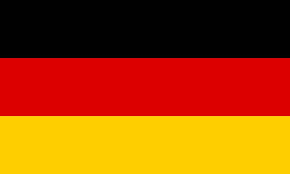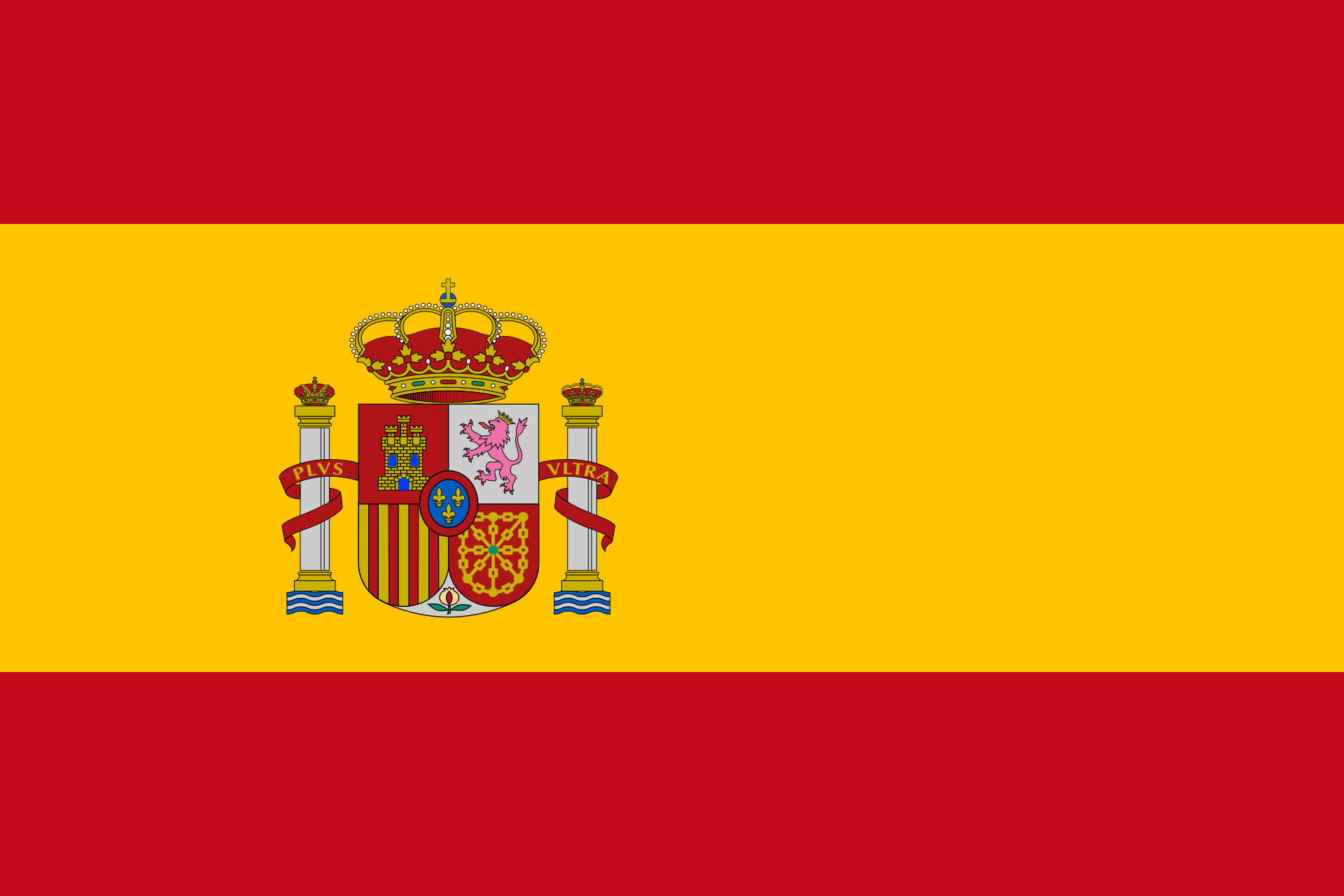# Transforming plane equationsEnter one of the three plane equations. Mathepower calculates the other ones.
Choose how the plane is given.
• parametric equation:

 E: x =+ r+ s• Coordinate form:

• Point-normal form:

 E: (x-)⋅=0
• Given through three points